# Build a Pivot Table in Excel VBA

Keep in mind that this chapter is not meant to imply that you use VBA to build pivot tables to give to your users! Rather, its purpose is to remind you that pivot tables can be used as a means to an end; you can use a pivot table to extract a summary of data and then use that summary elsewhere.

TIP

The code listings from this chapter are available for download at http://www.MrExcel.com/pivotbookdata.html.

In Excel 2000 and newer, you first build a pivot cache object to describe the input area of the data:

` Dim WSD As Worksheet Dim PTCache As PivotCache Dim PT As PivotTable Dim PRange As Range Dim FinalRow As Long Dim FinalCol As Long Set WSD = Worksheets("PivotTable") ' Delete any prior pivot tables For Each PT In WSD.PivotTables     PT.TableRange2.Clear Next PT ' Define input area and set up a Pivot Cache FinalRow = WSD.Cells(Application.Rows.Count, 1).End(xlUp).Row FinalCol = WSD.Cells(1, Application.Columns.Count).End(xlToLeft).Column Set PRange = WSD.Cells(1, 1).Resize(FinalRow, FinalCol) Set PTCache = ActiveWorkbook.PivotCaches.Add(SourceType:=xlDatabase, _     SourceData:=PRange) `

After the pivot cache is defined, use the CreatePivotTable method to create a blank pivot table based on the defined pivot cache:

` Set PT = PTCache.CreatePivotTable(TableDestination:=WSD.Cells(2, FinalCol + 2), _     TableName:="PivotTable1") `

In the CreatePivotTable method, you specify the output location and optionally give the table a name. After running this line of code, you have a strange-looking blank pivot table, like the one shown in Figure 12.2.

##### Figure 12.2. Immediately after you use the CreatePivotTable method, Excel gives you a four-cell blank pivot table that is not very useful. You now have to use code to drop fields onto the table.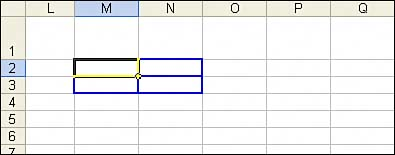If you are using the Layout dialog box in the user interface to build the pivot table, Excel does not recalculate the pivot table after you drop each field onto the table. By default in VBA, Excel calculates the pivot table as you execute each step of building the table. This could require the pivot table to be executed a half-dozen times before you get to the final result. To speed up your code execution, you can temporarily turn off calculation of the pivot table by using the ManualUpdate property:

` PT.ManualUpdate = True `

You can now run through the steps needed to lay out the pivot table. In the .AddFields method, you can specify one or more fields that should be in the row, column, or page area of the pivot table:

` ' Set up the row & column fields PT.AddFields RowFields:=Array("Line of Business", "Model"), _     ColumnFields:="Region" `

To add a field such as Revenue to the data area of the table, you change the Orientation property of the field to be xlDataField.

#### Getting a Sum Instead of a Count

Excel is smart. When you build a report with revenue, it assumes you want to sum the revenue. But, there is a problem. Say that one of the revenue cells is accidentally blank. When you build the pivot table, even though 99.9% of fields are numeric, Excel assumes you have alphanumeric data and offers to count this field. This is annoying. It seems to be an anomaly that on one hand, you are expected to make sure that 100% of your cells have numeric data, but on the other hand, the results of the pivot table are often filled with non-numeric blank cells.

When you build the pivot table in the Excel interface, you should take care in the Layout dialog box to notice that the field reads Count of Revenue instead of Sum of Revenue. At that point, the right thing is to go back and fix the data, but what people usually do is double-click the Count of Revenue button and change it to Sum of Revenue.

In VBA, you should always explicitly define that you are creating a sum of revenue by explicitly setting the Function property to xlSum:

` ' Set up the data fields With PT.PivotFields("Revenue")     .Orientation = xlDataField     .Function = xlSum     .Position = 1 End With `

At this point, you've given VBA all the settings required to correctly generate the pivot table. If you set ManualUpdate to False, Excel calculates and draws the pivot table. You can immediately thereafter set this back to TRue:

` ' Calc the pivot table PT.ManualUpdate = False PT.ManualUpdate = True `

At this point, you will have a complete pivot table like the one shown in Figure 12.3.

##### Figure 12.3. Less than 50 lines of code create this pivot table in under a second.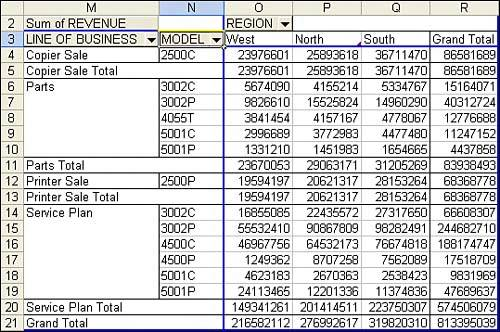Here is the complete code used to generate the pivot table:

` Sub CreatePivot()     Dim WSD As Worksheet     Dim PTCache As PivotCache     Dim PT As PivotTable     Dim PRange As Range     Dim FinalRow As Long     Dim FinalCol As Long     Set WSD = Worksheets("PivotTable")     ' Delete any prior pivot tables     For Each PT In WSD.PivotTables         PT.TableRange2.Clear     Next PT     ' Define input area and set up a Pivot Cache     FinalRow = WSD.Cells(Application.Rows.Count, 1).End(xlUp).Row     FinalCol = WSD.Cells(1, Application.Columns.Count). _         End(xlToLeft).Column     Set PRange = WSD.Cells(1, 1).Resize(FinalRow, FinalCol)     Set PTCache = ActiveWorkbook.PivotCaches.Add(SourceType:= _         xlDatabase, SourceData:=PRange)     ' Create the Pivot Table from the Pivot Cache     Set PT = PTCache.CreatePivotTable(TableDestination:=WSD. _         Cells(2, FinalCol + 2), TableName:="PivotTable1")     ' Turn off updating while building the table     PT.ManualUpdate = True     ' Set up the row & column fields     PT.AddFields RowFields:=Array("Line of Business", "Model"), _         ColumnFields:="Region"     ' Set up the data fields     With PT.PivotFields("Revenue")         .Orientation = xlDataField         .Function = xlSum         .Position = 1     End With     ' Calc the pivot table     PT.ManualUpdate = False     PT.ManualUpdate = True End Sub `

#### Cannot Move or Change Part of a Pivot Report

Although pivot tables are incredible, they have annoying limitations. You cannot move or change just a part of a pivot table. For example, try to run a macro that would delete column R, which contains the Grand Total column of the pivot table. The macro comes to a screeching halt with an error 1004, as shown in Figure 12.4.

##### Figure 12.4. You cannot delete just a part of a pivot table. To get around this limitation, you can change the summary from a pivot table to just values.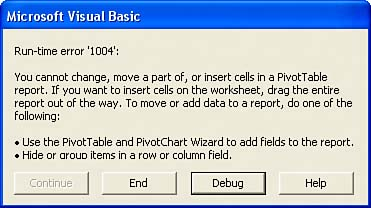#### Size of a Finished Pivot Table

It is difficult to know the size of a pivot table in advance. If you run a report of transactional data on one day, you may or may not have sales from the West region, for example. This could cause your table to be either five or six columns wide. Therefore, you should use the special property TableRange2 to refer to the entire resultant pivot table.

Because of the limitations of pivot tables, you should generally copy the results of a pivot table to a new location on the worksheet and then delete the original pivot table. The code in CreateSummaryReportUsingPivot() creates a small pivot table. Note that you can set the ColumnGrand and RowGrand properties of the table to False to prevent the totals from being added to the table.

PT.TableRange2 includes the entire pivot table. In this case, this includes the extra row at the top with the button Sum of Revenue. To eliminate that row, the code copies PT.TableRange2, but offsets this selection by one row by using .Offset(1, 0). Depending on the nature of your pivot table, you might need to use an offset of two or more rows to get rid of extraneous information at the top of the pivot table.

The code copies PT.TableRange2 and does a PasteSpecial to a cell three rows below the current pivot table. At that point in the code, your worksheet appears as shown in Figure 12.5. The table in M2 is a live pivot table, and the table in M16 is just the copied results.

##### Figure 12.5. An intermediate result of the macro. Only the summary in M16:P25 will remain after the macro finishes.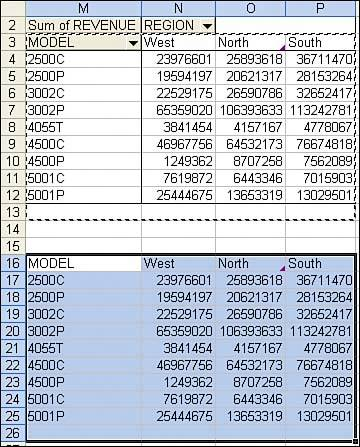You can then totally eliminate the pivot table by applying the Clear method to the entire table. If your code is then going on to do additional formatting, you should remove the pivot cache from memory by setting PTCache equal to Nothing:

` Sub CreateSummaryReportUsingPivot()     ' Use a Pivot Table to create a static summary report     ' with model going down the rows and regions across     Dim WSD As Worksheet     Dim PTCache As PivotCache     Dim PT As PivotTable     Dim PRange As Range     Dim FinalRow As Long     Dim FinalCol As Long     Set WSD = Worksheets("PivotTable")     ' Delete any prior pivot tables     For Each PT In WSD.PivotTables         PT.TableRange2.Clear     Next PT     ' Define input area and set up a Pivot Cache     FinalRow = WSD.Cells(Application.Rows.Count, 1).End(xlUp).Row     FinalCol = WSD.Cells(1, Application.Columns.Count). _         End(xlToLeft).Column     Set PRange = WSD.Cells(1, 1).Resize(FinalRow, FinalCol)     Set PTCache = ActiveWorkbook.PivotCaches.Add(SourceType:= _         xlDatabase, SourceData:=PRange)     ' Create the Pivot Table from the Pivot Cache     Set PT = PTCache.CreatePivotTable(TableDestination:=WSD. _         Cells(2, FinalCol + 2), TableName:="PivotTable1")     ' Turn off updating while building the table     PT.ManualUpdate = True     ' Set up the row fields     PT.AddFields RowFields:="Model", ColumnFields:="Region"     ' Set up the data fields     With PT.PivotFields("Revenue")         .Orientation = xlDataField         .Function = xlSum         .Position = 1     End With     With PT         .ColumnGrand = False         .RowGrand = False         .NullString = "0"     End With     ' Calc the pivot table     PT.ManualUpdate = False     PT.ManualUpdate = True     ' PT.TableRange2 contains the results. Move these to J10     ' as just values and not a real pivot table.     PT.TableRange2.Offset(1, 0).Copy     WSD.Cells(5 + PT.TableRange2.Rows.Count, FinalCol + 2). _         PasteSpecial xlPasteValues     ' At this point, the worksheet looks like Figure 12.5     ' Delete the original Pivot Table & the Pivot Cache     PT.TableRange2.Clear     Set PTCache = Nothing End Sub `

The preceding code will create the pivot table. It then copies the results as values and pastes them as values in M16:P25. Figure 12.5 shows an intermediate result just before the original pivot table is cleared.

So far, you've walked through building the simplest of pivot table reports. Pivot tables offer far more flexibility. Read on for more complex reporting examples.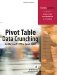Pivot Table Data Crunching for Microsoft Office Excel 2007
ISBN: 0789736012
EAN: 2147483647
Year: 2003
Pages: 140

Similar book on Amazon